and ortutorials

Basics

Radiometry is the field of photonics describing and quantifying light energy emitted by a source, received by a surface, transmitted through a medium or through an optical system.

Among all the parameters used in radiometry, an important one is the "solid angle". It's a mathematical entity representing an angle in three dimensions.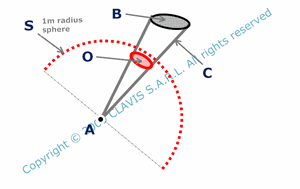Considering a point A and an object B, C is the cone defined by the lines from A and passing through the outline of the object B. S is the sphere of center A and with a 1 meter radius. The angle solid O defined by the cone C is the area of the intersection of S with C. It is expressed in steradians ( sr ).

There are some other specific parameters currently used in radiometry.

The flux ( F or P ) represents a considered optical power. It can be emitted by a light source, transmitted by an optical system, detected by a photodetector, etc

The intensity ( I ) of a light source represents the flux emitted in a given direction. It's the derived function of the flux by solid angle. It's expressed in W/sr.

The luminance or radiance( L ) is the density of intensity emitted by a light source. It's the derived function of the intensity by source area. It is a local parameter expressed in W/sr/m2.

The emittance ( Em ) is the flux density emitted by a light source. It's the derived function of the flux by source area. It is a local parameter expressed in W/m2.

The illuminance or irradiance ( E ) of an object ( screen, photodetector, camera,) is the flux density received by the object. It's the derived function of received flux by object area. It's a local parameter expressed in W/m2.

The optical power is generally expressed in radiometric unit ( Watts ) which is not adapted to light perception by the human eye. One particularity of the human eye is to have a wavelength depending sensitivity. For example, 1 watt in the green-yellow at 555 nm ( maximum diurnal eye sensitivity ) is seen brighter than 1 Watt at in the red at 650 nm. It's then useful to take into account this characteristic. The photometry is the part of photonic science describing and quantifying light energy as seen by the human eye. Photometry uses its own units like the "lumen" which is a power unit depending on wavelength. The curve giving the ratio Lumen/Watt vs wavelength is homotetic to the diurnal sensitivity of the human eye. When normalized, this curve represents the luminous efficiency of the human eye at each wavelength for the diurnal vision. Lumen is said to be a "photometric" power unit. Derived parameters like intensity, radiance ( or luminance ), irradiance ( or illuminance), emittance can also be expressed in photometric units. Usually, the words luminance and illuminance are used in photometry and are expressed with photometric units while the words radiance and irradiance are used in radiometry and are expressed in radiometric units.

So far, except in some conversion pages, only radiometric units are used in the calculation pages.

Sources

There are mainly two kinds of light sources ( laser non included ). Thermal light sources transform part of their thermal energy into light. They have a continuous spectrum and can generally be modelized with black body theory. Examples of thermal sources include sunlight, tungsten light sources, etc...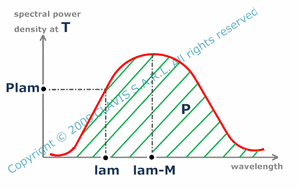For this kind of light sources, the spectrum depends only on the temperature ( T ). Consequently, knowing T allows to calculate the optical power density ( P ), the spectral power density ( Plam ), which is the derived function of the power density by wavelength, and the wavelength ( lam-M ) giving the maximum spectral power density.

In a luminescent light source, an atom emits a photon when loosing energy by collapsing from a "high energy" state ( excited state ) to a "low energy" state. The energy gap defines the photon energy and consequently its wavelength. As atoms have discrete energy gaps, a luminescent light source produces a discontinuous spectrum made of emission lines. Naturally, atoms are mostly in the "low energy" state. Light is emitted only if atoms have been excited first. The excitation can be electrical, optical, acoustical, mechanical, etc, depending on the light source. Examples of luminescent light sources include Mercury-vapor lamps and sodium lamps, etc...

For luminescent light sources, FWHM ( full width half maximum ) on the global spectrum makes no sens but can be defined for each particular emission line.

In the general case, light sources parameters may be tricky to calculate as emitting surfaces may have complicated shapes and complex intensity/ luminance distributions. Two simple models are often used. The first one is the isotropic source which intensity I is constant whatever the considered direction.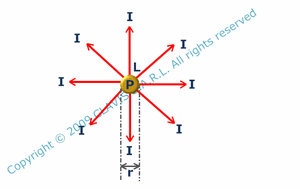Generally, isotropic sources are ponctual or spherical. The total emitted flux ( or optical power P ) is a function of the intensity ( I ). For spherical sources, as I is a function of the radiance L and the source radius r, P can be calculated with r and L. Knowing the light source power in radiometric units ( P ) and the luminous efficiency ( K ) allows to calculate the intensity ( I ) in photometric units.

The second model concerns lambertian sources which are sources with a constant radiance whatever the considered source element and the observation direction.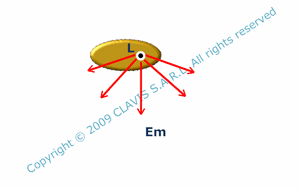The emittance ( Em ) of a lambertian source depends only on its radiance ( L ).When a plane circular lambertian source is at infinity, the irradiance of a surface on its axis depends on the angular size ( teta ) and the radiance ( L ) of the source but also on the surface inclination ( i ) with the light source axis. The calculation is approached. It is assumed that teta is small.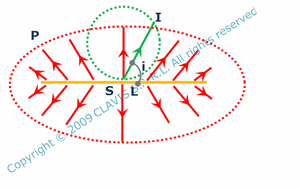For a plane circular lambertian source, the flux ( or optical power P ) is a function of the radiance ( L ) and the surface area ( S ). The intensity ( I ) depends on L and S but also on the observation angle ( i ).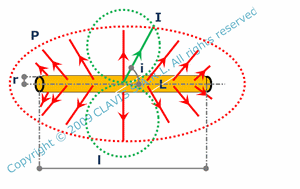For a cylindrical lambertian source, the flux ( P ) is a function of the radius ( r ), the length ( l ) and the radiance ( L ). The intensity ( I ) depends on r, l and L but also on the observation angle ( i ).

Systems

A radiometric ( or photometric ) system includes at least a light source illuminating a target ( detector, screen, eye,).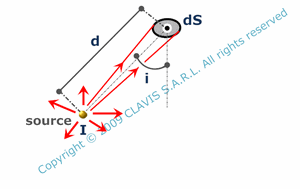A basic calculation concerns the luminous flux ( F ) emitted by a ponctual source and received by a surface element ( dS ). F depends on the intensity ( I ) in the illumination direction, the distance from the source to the surface element ( d ) and the angle ( i ) between the normal to the surface element and the illumination direction. The calculation is approached. It is assumed that the surface element dS is small regarding d.

Sometimes, an optical system can be added to increase the amount of light on the target. When it's the case, the calculations assume that Gauss conditions are respected.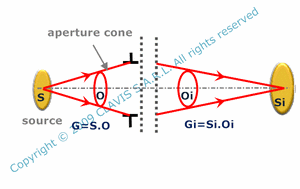An important parameter is then the geometrical extent G. G is the product of the light source area ( supposed plane and perpendicular to the optical axis ) by the solid angle defined by the aperture cone. For small lambertian light sources, G is proportionnal to the luminous flux transmitted through the optical system. Consequently, in Gauss conditions, the geometrical extent is unvariant, which means that G = Gi, Gi being the geometrical extent in the image space.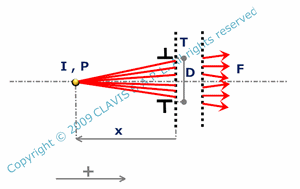The luminous flux ( F ) emitted by an isotropic light source and going through an optical system depends on the total optical power ( P ) or its intensity ( I ) of the light source, the aperture diameter ( D ), the distance from the primary principal plane to the source ( x ) and the transmission ratio ( T ) of the optical system.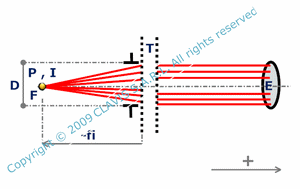When a ponctual isotropic light source is located at the front focal point of an optical system, the irradiance ( E ) of the lightspot on a plane screen perpendicular to the optical axis is a function of the total optical power ( P ) or intensity of the light source ( I ), the aperture diameter ( D ), the focal length ( fi ) and the transmission ratio of the optical system ( T ).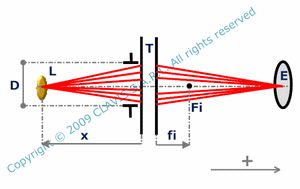The luminous flux ( F ) emitted by a small lambertian light source and going through an optical system as well as the irradiance ( E ) in the conjugated plane depend on the radiance ( L ) of the light source, the aperture diameter ( D ), the distance ( x ) from the front principal plane to the source and the transmission ratio ( T ) and the source area ( only for the transmitted flux ). The calculation are approached. It is assumed that the light source and D are small regarding x.For a lambertian light source located at infinity, the irradiance ( Es) of the light spot in the back focal plane of an optical system depends on the radiance ( L ), the aperture diameter ( D ), the focal length ( fi ) and the transmission ratio ( T ). The calculation is approached and it is assumed that the angular size of the object is small ( which means that the dimensions of its image in the focal plane are small regarding fi ). It is also assumed that D is much smaller than fi. When the aperture number ( N ) is given instead of D, the irradiance ( Es ) depends on L, N and T. The gain of irradiance (ratio of irradiance through the optical system on the irradiance produced by direct illumination ) depends also on the angular size ( teta ) of the source.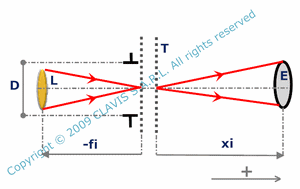When a small lambertian source is in the front focal plane of a slightly opened optical system and illuminates a screen ( perpendicular to the optical axis ), the irradiance ( E ) of the lightened part of the screen depends on the radiance ( L ), the aperture diameter ( D ), the transmission ratio ( T ) and the distance ( xi ) from the secondary principal plane to the screen. The calculation is approached. It is assumed that the object and D are small regarding fi and xi.When a light source illuminates directly a photodetector, the photocurrent ( Iph ) is a function of the detector sensitivity ( s ) and the detector area ( Sph ), the distance from the source to the detector ( d ) and the source intensity ( I ). In the case of a lambertian source, Iph depends on s, Sph, d, radiance ( L ) and source area ( Ss ).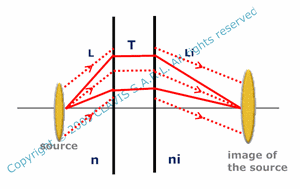It is possible to calculate the radiance ( Li ) of a source conjugated of a primary source ( with a radiance L ) by an optical system. If refraction indexes are the same in the object and image spaces and that the transmission ratio is 1, L = Li. Otherwise, Li depends on L, the transmission ratio ( T ) and refraction indexes ( n and ni ) of object and image spaces.

Diffusion

Diffusing materials illuminated by a light source ( primary source ) may behave as a secondary source.Supposing that the diffusion is perfect ( isotropic ), it's possible to calculate the secondary source radiance ( L ), knowing its illuminance ( E ) by the primary source. If its surface is plane, it is also possible to calculate the secondary source intensity ( I ), knowing the luminous flux ( F ) received by the diffusing surface and the angle of observation ( i ) with the normal to the surface.

References

"Cours de photométrie radiométrie" - Ecole Supérieure d'optique - 1987 - author : F.Desvignes.

"Optique géométrique Imagerie et instruments" - 2007 - author : Bernard Balland.

"cours de radiométrie et détection optique" - author : Jean Louis Meyzonette.